Skip to main content Accessibility help
Home
Hostname: page-component-747cfc64b6-db5sh Total loading time: 0.223 Render date: 2021-06-18T07:03:04.498Z Has data issue: true Feature Flags: { "shouldUseShareProductTool": true, "shouldUseHypothesis": true, "isUnsiloEnabled": true, "metricsAbstractViews": false, "figures": true, "newCiteModal": false, "newCitedByModal": true, "newEcommerce": true }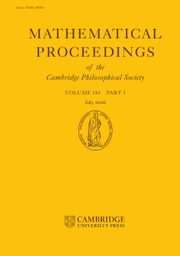Mathematical Proceedings of the Cambridge Philosophical Society

# On the quotients of mapping class groups of surfaces by the Johnson subgroups

Published online by Cambridge University Press:  27 November 2019

## Abstract

We study quotients of mapping class groups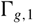${\Gamma _{g,1}}$ of oriented surfaces with one boundary component by the subgroups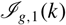${{\cal I}_{g,1}}(k)$ in the Johnson filtrations, and we show that the stable classifying spaces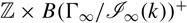${\mathbb {Z}} \times B{({\Gamma _\infty }/{{\cal I}_\infty }(k))^ + }$ after plus-construction are infinite loop spaces, fitting into a tower of infinite loop space maps that interpolates between the infinite loop spaces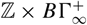${\mathbb {Z}} \times B\Gamma _\infty ^ +$ and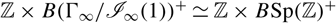${\mathbb {Z}} \times B{({\Gamma _\infty }/{{\cal I}_\infty }(1))^ + } \simeq {\mathbb {Z}} \times B{\rm{Sp}}{({\mathbb {Z}})^ + }$ . We also show that for each level k of the Johnson filtration, the homology of these quotients with suitable systems of twisted coefficients stabilises as the genus of the surface goes to infinity.

## MSC classification

Type
Research Article
Information
Copyright
© Cambridge Philosophical Society 2019

## Access options

Get access to the full version of this content by using one of the access options below.

## References

Andreadakis, S.. On the automorphisms of free groups and free nilpotent groups. Proc. London Math. Soc. 3.15 (1965), pp. 239268.CrossRefGoogle Scholar
Basterra, M., Bobkova, I., Ponto, K., Tillmann, U. and Yeakel, S.. Infinite loop spaces from operads with homological stability. Adv. Math. 321 (2017), pp. 391430.CrossRefGoogle Scholar
Borel, A.. Stable real cohomology of arithmetic groups. Ann. Sci. École Norm. Sup. (4), 7 (1974), 235272.CrossRefGoogle Scholar
Borel, A.. Stable real cohomology of arithmetic groups II. Manifolds and Lie groups: papers in honor of Yozo Matsushima. Progr. Math. 14 (Birkhäuser, 1981), pp. 2155.CrossRefGoogle Scholar
Brown, K. S.. Cohomology of groups. Graduate Texts in Mathematics 87 (Springer-Verlag, 1982).CrossRefGoogle Scholar
Charney, R.. A generalization of a theorem of Vogtmann. Pure Appl. Alg. 44.1 (1987), pp. 107125.CrossRefGoogle Scholar
Church, T. and Ellenberg, J. S.. Homology of FI-modules. Geom. Topol. 21.4 (2017), pp. 23732418.CrossRefGoogle Scholar
Church, T., Ellenberg, J. S. and Farb, B.. FI-modules and stability for representations of symmetric groups. Duke Math. J. 164.9 (2015), pp. 18331910.CrossRefGoogle Scholar
Church, T., Ellenberg, J. S., Farb, B. and Nagpal, R.. FI-modules over Noetherian rings. Geom. Topol. 18.5 (2014), pp. 29512984.CrossRefGoogle Scholar
Church, T. and Putman, A.. Generating the Johnson filtration. Geom. Topol. 19.4 (2015), pp. 22172255.CrossRefGoogle Scholar
Dehn, M.. Papers on Group Theory and Topology. Trans. German by John Stillwell (Springer-Verlag, 1987).CrossRefGoogle Scholar
Farb, B. and Margalit, D.. A primer on mapping class groups. Princeton Mathematical Series 49 (Princeton University Press, 2012).CrossRefGoogle Scholar
Harer, J. L.. Stability of the homology of the mapping class groups of orientable surfaces. Ann. of Math. (2) 121.2 (1985), pp. 215249.CrossRefGoogle Scholar
Johnson, D.. A survey of the torelli group. Low-dimensional topology (Proceedings of the Special Session held at the 87th Annual Meeting of the American Mathematical Society, San Francisco, California, January 1981). Ed. by Samuel, J. Lomonaco Jr Contemp. Math. 20 (1983).Google Scholar
Johnson, D.. The structure of the torelli group—II: a characterization of the group generated by twists on bounding curves. Topology 24.2 (1985), pp. 113126.CrossRefGoogle Scholar
Kawazumi, N. and Morita, S.. The primary approximation to the cohomology of the moduli space of curves and cocycles for the stable characteristic classes. Math. Res. Lett. 3.5 (1996), 629641.CrossRefGoogle Scholar
Mac Lane, S.. Categorical algebra. Bull. Amer. Math. Soc. 71.1 (1965).CrossRefGoogle Scholar
Madsen, I. and Weiss, M.. The stable moduli space of Riemann surfaces: Mumford’s conjecture. Ann. of Math. (2) 165.3 (2007), pp. 843941.CrossRefGoogle Scholar
McDuff, D. and Segal, G.. Homology fibrations and the “group-completion” theorem. Invent. Math. 31 (1976), pp. 279284.CrossRefGoogle Scholar
Patzt, P.. Representation stability for filtrations of torelli groups. Math. Ann. 372 (1–2) (2018), pp. 257298.CrossRefGoogle Scholar
Putman, A. and Sam, S.. Representation stability and finite linear groups. Duke Math. J. 166.13 (2017), pp. 25212598.CrossRefGoogle Scholar
Randal–Williams, O. and Wahl, N.. Homological stability for automorphism groups. Adv. Math. 318 (2017), pp. 534626.CrossRefGoogle Scholar
Serre, J.-P.. Lie algebras and Lie groups. Lecture Notes in Math. 1500 (Springer, 2006).Google Scholar
Szymik, M.. The rational stable homology of mapping class groups of universal nil-manifolds. To appear in Annales de l’Institut Fourier; arXiv:1605.06508 (2016).Google Scholar
Szymik, M.. Twisted homological stability for extensions and automorphism groups of free nilpotent groups. Journal of K-theory: K-theory and its Applications to Algebra, Geometry and Topology, 14.1 (2014), pp. 185201.CrossRefGoogle Scholar
Tillmann, U.. Higher genus surface operad detects infinite loop spaces. Math. Ann. 317.3 (2000), pp. 613628.CrossRefGoogle Scholar
Tillmann, U.. On the homotopy of the stable mapping class group. Invent. Math. 130.2 (1997), pp. 257275.CrossRefGoogle Scholar
Wahl, N.. From mapping class groups to automorphism groups of free groups. J. London Math. Soc. (2) 72.2 (2005), pp. 510524.CrossRefGoogle Scholar
Wahl, N.. Infinite loop space structure(s) on the stable mapping class group. Topology 43.2 (2004), pp. 343368.CrossRefGoogle Scholar
Weibel, C.. An introduction to homological algebra. Camb. Stud. Adv. Math. 38 (Cambridge University Press, 1994).CrossRefGoogle Scholar
Zeman, T.. Operads with homological stability and infinite loop space structures. In preparation.Google Scholar

# Send article to Kindle

To send this article to your Kindle, first ensure no-reply@cambridge.org is added to your Approved Personal Document E-mail List under your Personal Document Settings on the Manage Your Content and Devices page of your Amazon account. Then enter the ‘name’ part of your Kindle email address below. Find out more about sending to your Kindle. Find out more about sending to your Kindle.

Note you can select to send to either the @free.kindle.com or @kindle.com variations. ‘@free.kindle.com’ emails are free but can only be sent to your device when it is connected to wi-fi. ‘@kindle.com’ emails can be delivered even when you are not connected to wi-fi, but note that service fees apply.

Find out more about the Kindle Personal Document Service.

On the quotients of mapping class groups of surfaces by the Johnson subgroups
Available formats
×

# Send article to Dropbox

To send this article to your Dropbox account, please select one or more formats and confirm that you agree to abide by our usage policies. If this is the first time you use this feature, you will be asked to authorise Cambridge Core to connect with your <service> account. Find out more about sending content to Dropbox.

On the quotients of mapping class groups of surfaces by the Johnson subgroups
Available formats
×

# Send article to Google Drive

To send this article to your Google Drive account, please select one or more formats and confirm that you agree to abide by our usage policies. If this is the first time you use this feature, you will be asked to authorise Cambridge Core to connect with your <service> account. Find out more about sending content to Google Drive.

On the quotients of mapping class groups of surfaces by the Johnson subgroups
Available formats
×
×

#### Reply to:Submit a response

Please enter your response.

#### Your details

Please enter a valid email address.

#### Conflicting interests

Do you have any conflicting interests? *